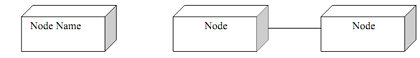## Define the node of object oriented modeling, Computer Engineering

Assignment Help:

Define the node of object oriented modeling

A node is a physical element which exists at runtime and represents a computational resource usually having a large memory and often process capability. In UML, node is shown as a cube. The notation for the node is shown as:#### Bit manipulation techniques and mathematical functions, Within micro contro...

Within micro controller's software, it is very useful to be able to manipulate binary bits i.e. from ports etc. The ALU has command to shift data, rotate data, compare data, set/cl

#### By which the excess-3 code of decimal 7 is represented , The excess-3 code ...

The excess-3 code of decimal 7 is represented by ? Ans. An excess 3 code of decimal 7 is equal to the binary code +3.

#### Minimum possibility -minimax algorithm, Minimum possibility -minimax algori...

Minimum possibility -minimax algorithm: Finally, we want to put the scores on the top edges in the tree. So there is over again a choice. Whenever, in this case, we have to r

#### What is testing, What is testing? List its types. Testing ensures that ...

What is testing? List its types. Testing ensures that the application is suitable for actual use and that it truthfully satisfies the requirements. Types are: Unit te

#### COMPUTER GRAPHICS, what is mean by refersing screen

what is mean by refersing screen

#### How many input ANDand OR gates are required, How many two input AND gates a...

How many two input AND gates and two input OR gates are required to realize Y = BD+CE+AB ? Ans. Here three product terms, therefore three AND gates of two inputs are needed.

#### what is code for adding two integers in java?, import java.util.Scanner;  ...

import java.util.Scanner;   class AddNumbers {    public static void main(String args[])    {       int x, y, z;       System.out.println("Enter two integers to calculate their sum

#### Compute the different stages of kohlbergs theory, Problem 1 (a) Identi...

Problem 1 (a) Identify and briefly describe the possible roles of Codes of Ethics (b) Describe why is a code of ethics important to stakeholders. (c) Explain how should

#### Define abap/4 layer, Define ABAP/4 layer? The ABAP/4 layer defines the ...

Define ABAP/4 layer? The ABAP/4 layer defines the data formats used by the ABAP/4 processor.

#### Explain simple network management protocol, Explain SNMP (simple network ma...

Explain SNMP (simple network management protocol). Once SNMP is used the management station sends a request to an agent asking this for commanding or information this to update